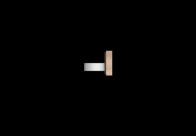## Monday, December 2, 2013

### Independent Friction Labs and Another Capstone Project

I have my Honors Physics students prepare small electronic posters for their final lab of the first term, the Independent Friction Lab. In this lab, students have to come up with an experiment, make an informal proposal, execute the experiment, and analyze the results.

The experiment really just needs to have something to do with friction, and I get a wide variety of them. I have them create a single PP or similar slide, sized 24"x18"; they're great to print at Staples. I ask them to email me a draft a day or so before the presentations, and they present the revised projects.
Here are a few of this year's experiments:

This group used a Pasco friction cart; they let it slide on a cart track, used the velocity graph to determine the coefficient of kinetic friction, and then determined the hanging mass that would pull the friction cart at constant speed (verifying that with another motion detector graph).

This group found the coefficient of static friction between a block and a ramp in a neat way: they used a half-Atwood setup, changing the mass until the block slipped, but performed that experiment at several angles. They then predicted a function for that maximum mass as a function of the block's known mass, the angle, and the unknown friction coefficient. Graphing their data, Logger Pro found the static friction coefficient by regression, providing both a quality value and confirmation of the model that they used to describe the situation.

These students compared the effective coefficients of friction for a ball rolling (without slipping) and the same ball under backspin (backspin persists until it turns around). They're essentially determining the coefficients of rolling and kinetic friction, showing that the kinetic friction coefficient's much larger.

This one seems to come up every year, and it's always fun. They used video analysis to determine the coefficient of kinetic friction between socks and several surfaces. The tricky unseen part is the big possible variation in normal force from foot to foot and moment to moment

A second AP Physics capstone project is also included here; the student was trying to model the interaction between a hockey stick and a puck. It ended up being a very difficult problem, but he gained some valuable ground and ended up with a functional scaled-back model.

Student work:

For my capstone project, I wanted to model the interaction between the blade of a hockey stick and the puck during a shot or pass in ice hockey. Using the ball and spring model of matter interactions, I created a VPython program where a constant force acts on the blade of the stick, but reverses direction at the center (0,0,0) to simulate the slowing down of the stick after reaching the midpoint where the x component of the force on the stick would be at its maximum. The force on the puck, however, does not follow the same constant pattern. Since materials act like springs with miniscule stretches, the force on the puck oscillates during the entire blade-puck interaction time even though the oscillation and resulting compression of the blade would be impossible to see with the naked eye. While this is not a perfect model since the blade remains at a constant angle, 90°, and the force magnitude remains constant in the direction of velocity and only changes direction by 180°, it does illustrate how matter interacts at the atomic scale. During the collision, both the force on the puck and the compression of the blade oscillate, but so slightly with the large spring constant that, looking at the velocity graph, the puck behaves like it would with a constant force and constant acceleration during contact.Screenshot at the moment of collisionGraphs of the "spring" compression, force exerted on the puck, and velocity of the puck as functions of time

from __future__ import division
from visual.graph import*
from visual import*

#create objects
h=.025
puck=cylinder(pos=(1,0,0),radius=0.038, height=h, axis=(0,h,0), mass=.17, velocity=vector(0,0,0))

l=.76
l=.02

stick=box(pos=beginpos, length=l, height=.076, width=0.3175, material=materials.wood, velocity=vector(0,0,0), mass=.7,)# axis=norm(R-beginpos)*.076, k=500000)

#R=vector(0,((2l)**2-stick.pos.x**2)**.5,)

scene.autoscale = False

#create forces

Fdirection=vector(-1,0,0)#norm(vector(-stick.axis.y, stick.axis.x,0))

Fmag=200

k= 10000#310575#414172.6

Fp=vector(k*s*Fdirection)

Fs=Fmag*Fdirection

#graph
gd =gdisplay(x=0, y=0, width=600, height=150, title='Fp vs. t', xtitle='t (s)', ytitle='Fp (N)', foreground=color.black, background=color.white, xmax=.25, xmin=0, ymax=100, ymin=-100)

Fpg=gcurve(color=color.red, gddisplay=gd)

gd2 =gdisplay(x=0, y=0, width=700, height=150, title='compression vs. t', xtitle='t (s)', ytitle='Compression (m)', foreground=color.black, background=color.white, xmax=.25, xmin=0, ymax=.01,ymin=-.005)

sg=gcurve(color=color.green, gdisplay=gd2)

vg = gdisplay(x=0, y=0, width=600, height=150, title='v vs. t', xtitle='t (s)', ytitle='Puck Velocity (m/s)',foreground=color.black, background=color.white, xmax=.25, xmin=0, ymax=0,ymin=-30)

vg=gcurve(color=color.blue, display=vg)

print s
#create loop

t=0
dt=.00001

while t<.25:

rate(10000)

if s>0 and stick.pos.x>0:

#stick

stick.velocity.x=stick.velocity.x+Fs.x/stick.mass*dt

stick.pos.x=stick.pos.x+stick.velocity.x*dt

#     R=vector(0,((2*l)**2-stick.pos.x**2)**.5,0)

#        stick.axis=norm(R-stick.pos)*stick.length

#puck

puck.velocity.x=puck.velocity.x+Fp.x/puck.mass*dt

puck.pos.x=puck.pos.x+puck.velocity.x*dt

#s

Fdirection=vector(-1,0,0)#norm(vector(-stick.axis.y, stick.axis.x,0))

Fp=vector(k*s*Fdirection)

Fs=Fmag*Fdirection-Fp

elif stick.pos.x>0:
#stick

stick.velocity.x=stick.velocity.x+Fs.x/stick.mass*dt

stick.pos.x=stick.pos.x+stick.velocity.x*dt

#        R=vector(0,((2*l)**2-stick.pos.x**2)**.5,0)

#        stick.axis=norm(R-stick.pos)*stick.length

#puck

puck.velocity.x=puck.velocity.x+Fp.x/puck.mass*dt

puck.pos.x=puck.pos.x+puck.velocity.x*dt

#s

Fdirection=vector(-1,0,0)#norm(vector(-stick.axis.y, stick.axis.x,0))
Fp=vector(k*s*Fdirection)

Fs=Fmag*Fdirection

elif stick.pos.x<0: abs="" arctan="" cos="" fdirection="vector(1,0,0)#norm(vector(-stick.axis.y," fp.x="" fp="vector(k*s*-Fdirection)" fs="Fmag*Fdirection" if="" puck.height="" puck.pos.x="puck.pos.x+puck.velocity.x*dt" puck.velocity.x="puck.velocity.x+Fp.x/puck.mass*dt" puck="" r.mag="" r="puck.pos+vector(puck.radius," s="stick.length/2-(stick.pos.x-puck.pos.x-puck.radius)" stick.axis.x="" stick.axis.y="" stick.axis="norm(R-stick.pos)*stick.length" stick.height="" stick.pos.x="stick.pos.x+stick.velocity.x*dt" stick.pos="" stick.velocity.x="stick.velocity.x+Fs.x/stick.mass*dt">0:
Fp.x=0

if stick.velocity.x>0:
stick.velocity.x=0

if s<0: abs="" arctan="" cos="" else:="" fp.x="" fpg.plot="" pos="(t," pre="" print="" puck.pos.x="puck.pos.x+puck.velocity.x*dt" puck.velocity.x="" puck.velocity="" r.mag="" s="" sg.plot="" stick.axis.x="" stick.axis.y="" stick.height="" stick.velocity.x="" t="t+dt" vg.plot="">